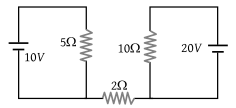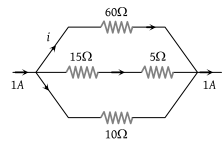A capacitor is connected to a cell of emf E having some internal resistance r. The potential difference across the

(1) Cell is < E

(2) Cell is E

(3) Capacitor is > E

(4) Capacitor is < E

Concept Questions :-

Grouping of cells
High Yielding Test Series + Question Bank - NEET 2020

Difficulty Level:

The maximum power drawn out of the cell from a source is given by (where r is internal resistance)

(1) ${E}^{2}/2r$

(2) ${E}^{2}/4r$

(3) ${E}^{2}/r$

(4) ${E}^{2}/3r$

Concept Questions :-

Heating effect of current
High Yielding Test Series + Question Bank - NEET 2020

Difficulty Level:

Find out the value of current through 2Ω resistance for the given circuit(1) 5 A

(2) 2 A

(3) Zero

(4) 4 A

Concept Questions :-

Kirchoff's voltage law
High Yielding Test Series + Question Bank - NEET 2020

Difficulty Level:

Two sources of equal emf are connected to an external resistance R. The internal resistances of the two sources are R1 and ${R}_{2}\text{\hspace{0.17em}}\left({R}_{2}>{R}_{1}\right)$. If the potential difference across the source having internal resistance R2 is zero, then

(1) $R={R}_{1}{R}_{2}/\left({R}_{1}+{R}_{2}\right)$

(2) $R={R}_{1}{R}_{2}/\left({R}_{2}-{R}_{1}\right)$

(3) $R={R}_{2}×\left({R}_{1}+{R}_{2}\right)/\left({R}_{2}-{R}_{1}\right)$

(4) $R={R}_{2}-{R}_{1}$

Concept Questions :-

Grouping of cells
High Yielding Test Series + Question Bank - NEET 2020

Difficulty Level:

The magnitude of i in ampere unit is(1) 0.1

(2) 0.3

(3) 0.6

(4) None of these

Concept Questions :-

Kirchoff's voltage law
High Yielding Test Series + Question Bank - NEET 2020

Difficulty Level:

To draw maximum current from a combination of cells, how should the cells be grouped

(1) Series

(2) Parallel

(3) Mixed

(4) Depends upon the relative values of external and internal resistance

Concept Questions :-

Grouping of cells
High Yielding Test Series + Question Bank - NEET 2020

Difficulty Level:

By ammeter, which of the following can be measured

(1) Electric potential

(2) Potential difference

(3) Current

(4) Resistance

Concept Questions :-

Current and current density
High Yielding Test Series + Question Bank - NEET 2020

Difficulty Level:

For measurement of potential difference, potentiometer is preferred in comparison to voltmeter because

(1) Potentiometer is more sensitive than voltmeter

(2) The resistance of potentiometer is less than voltmeter

(3) Potentiometer is cheaper than voltmeter

(4) Potentiometer does not take current from the circuit

Concept Questions :-

Meter bridge and potentiometer
High Yielding Test Series + Question Bank - NEET 2020

Difficulty Level:

The potential gradient along the length of a uniform wire is 10 volt/metre. B and C are the two points at 30 cm and 60 cm point on a meter scale fitted along the wire. The potential difference between B and C will be :

(1) 3 volt

(2) 0.4 volt

(3) 7 volt

(4) 4 volt

Concept Questions :-

Meter bridge and potentiometer
High Yielding Test Series + Question Bank - NEET 2020

Difficulty Level:

In Wheatstone's bridge P = 9 ohm, Q = 11 ohm, R = 4 ohm and S = 6 ohm. How much resistance must be put in parallel to the resistance S to balance the bridge

(1) 24 ohm

(2) $\frac{44}{9}$ohm

(3) 26.4 ohm

(4) 18.7 ohm

Concept Questions :-

Wheatstone bridge